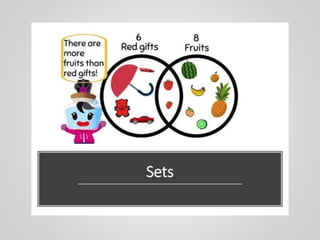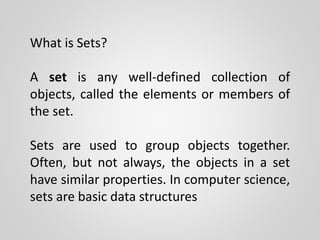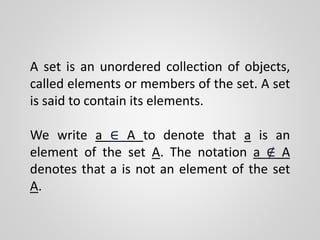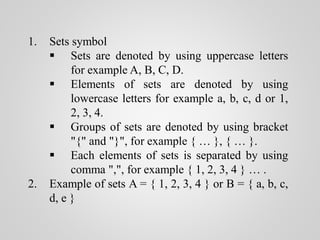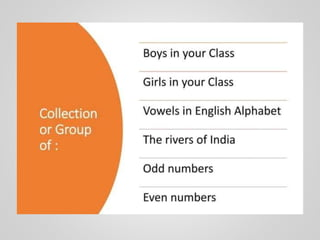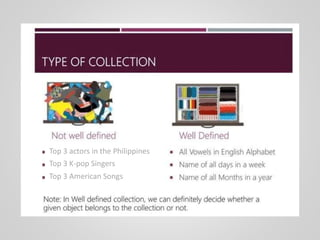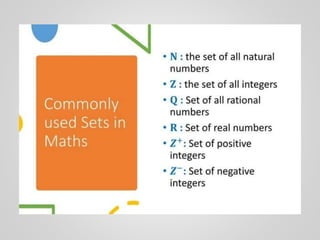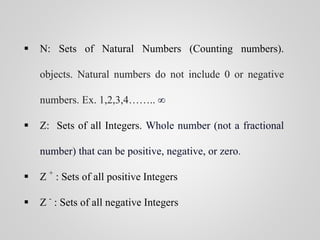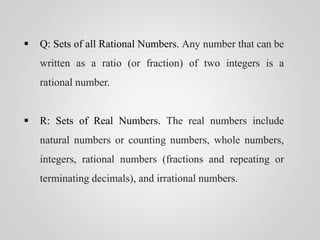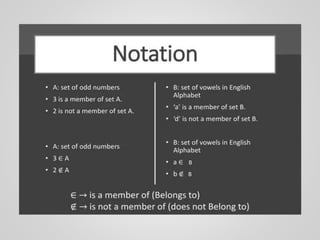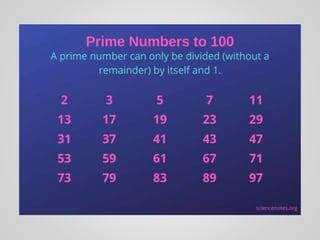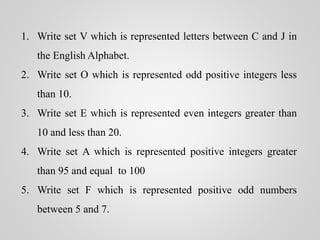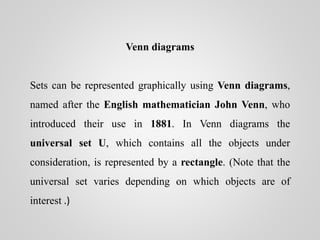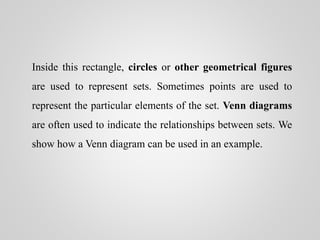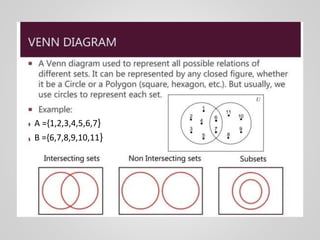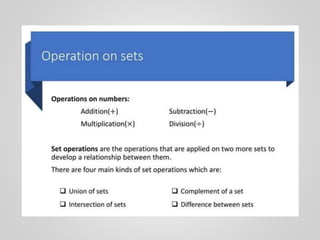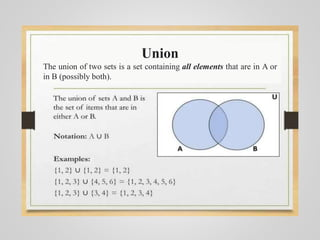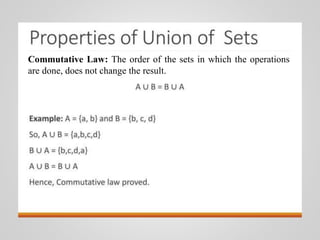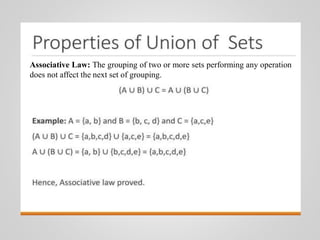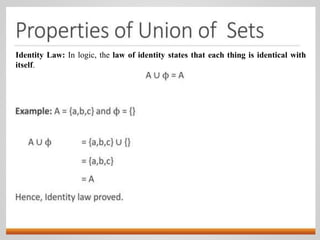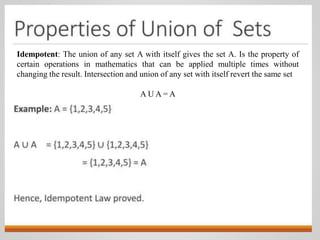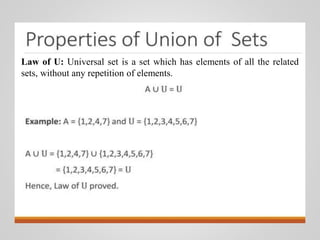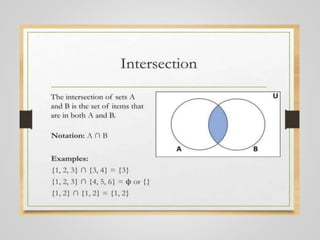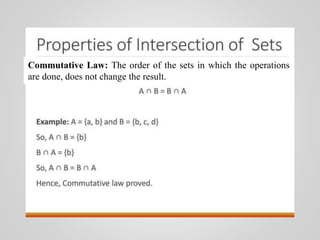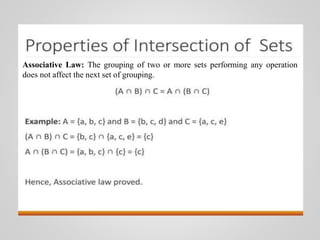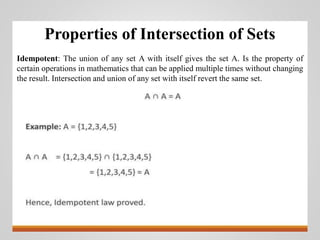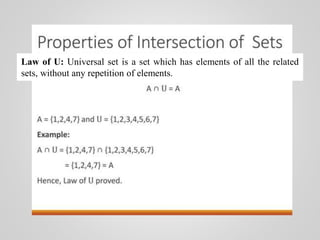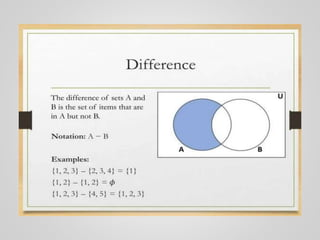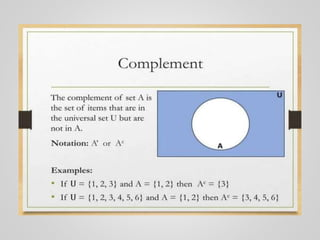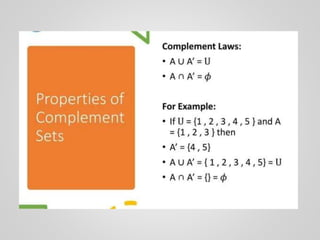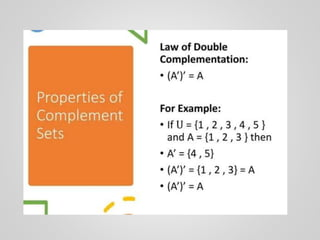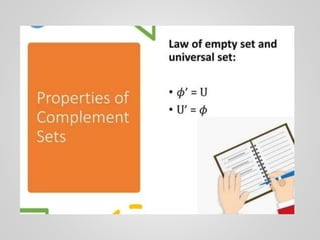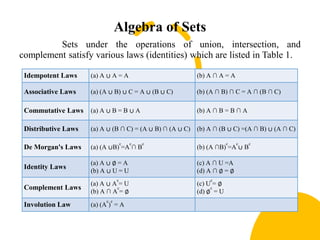1 of 35

### Sets.pptx

1. What is Sets? A set is any well-defined collection of objects, called the elements or members of the set. Sets are used to group objects together. Often, but not always, the objects in a set have similar properties. In computer science, sets are basic data structures
2. A set is an unordered collection of objects, called elements or members of the set. A set is said to contain its elements. We write a ∈ A to denote that a is an element of the set A. The notation a ∉ A denotes that a is not an element of the set A.
3. 1. Sets symbol  Sets are denoted by using uppercase letters for example A, B, C, D.  Elements of sets are denoted by using lowercase letters for example a, b, c, d or 1, 2, 3, 4.  Groups of sets are denoted by using bracket "{" and "}", for example { … }, { … }.  Each elements of sets is separated by using comma ",", for example { 1, 2, 3, 4 } … . 2. Example of sets A = { 1, 2, 3, 4 } or B = { a, b, c, d, e }
4. Top 3 actors in the Philippines Top 3 K-pop Singers Top 3 American Songs
5.  N: Sets of Natural Numbers (Counting numbers). objects. Natural numbers do not include 0 or negative numbers. Ex. 1,2,3,4…….. ∞  Z: Sets of all Integers. Whole number (not a fractional number) that can be positive, negative, or zero.  Z + : Sets of all positive Integers  Z - : Sets of all negative Integers
6.  Q: Sets of all Rational Numbers. Any number that can be written as a ratio (or fraction) of two integers is a rational number.  R: Sets of Real Numbers. The real numbers include natural numbers or counting numbers, whole numbers, integers, rational numbers (fractions and repeating or terminating decimals), and irrational numbers.
7. B B
8. The empty set There is a special set that has no elements. This set is called the empty set, or null set, and is denoted by ∅ . The empty set can also be denoted by { }. Often, a set of elements with certain properties turns out to be the null set. A: Set of prime numbers between 24 and 28 B: { }
9. 1. Write set V which is represented letters between C and J in the English Alphabet. 2. Write set O which is represented odd positive integers less than 10. 3. Write set E which is represented even integers greater than 10 and less than 20. 4. Write set A which is represented positive integers greater than 95 and equal to 100 5. Write set F which is represented positive odd numbers between 5 and 7.
10. Venn diagrams Sets can be represented graphically using Venn diagrams, named after the English mathematician John Venn, who introduced their use in 1881. In Venn diagrams the universal set U, which contains all the objects under consideration, is represented by a rectangle. (Note that the universal set varies depending on which objects are of interest .)
11. Inside this rectangle, circles or other geometrical figures are used to represent sets. Sometimes points are used to represent the particular elements of the set. Venn diagrams are often used to indicate the relationships between sets. We show how a Venn diagram can be used in an example.
12. A ={1,2,3,4,5,6,7} B ={6,7,8,9,10,11}
13. Union The union of two sets is a set containing all elements that are in A or in B (possibly both).
14. Commutative Law: The order of the sets in which the operations are done, does not change the result.
15. Associative Law: The grouping of two or more sets performing any operation does not affect the next set of grouping.
16. Identity Law: In logic, the law of identity states that each thing is identical with itself.
17. Idempotent: The union of any set A with itself gives the set A. Is the property of certain operations in mathematics that can be applied multiple times without changing the result. Intersection and union of any set with itself revert the same set A U A = A
18. Law of U: Universal set is a set which has elements of all the related sets, without any repetition of elements.
19. Commutative Law: The order of the sets in which the operations are done, does not change the result.
20. Associative Law: The grouping of two or more sets performing any operation does not affect the next set of grouping.
21. Properties of Intersection of Sets Idempotent: The union of any set A with itself gives the set A. Is the property of certain operations in mathematics that can be applied multiple times without changing the result. Intersection and union of any set with itself revert the same set.
22. Law of U: Universal set is a set which has elements of all the related sets, without any repetition of elements.
23. Algebra of Sets Sets under the operations of union, intersection, and complement satisfy various laws (identities) which are listed in Table 1. Idempotent Laws (a) A ∪ A = A (b) A ∩ A = A Associative Laws (a) (A ∪ B) ∪ C = A ∪ (B ∪ C) (b) (A ∩ B) ∩ C = A ∩ (B ∩ C) Commutative Laws (a) A ∪ B = B ∪ A (b) A ∩ B = B ∩ A Distributive Laws (a) A ∪ (B ∩ C) = (A ∪ B) ∩ (A ∪ C) (b) A ∩ (B ∪ C) =(A ∩ B) ∪ (A ∩ C) De Morgan's Laws (a) (A ∪B) c =A c ∩ B c (b) (A ∩B) c =A c ∪ B c Identity Laws (a) A ∪ ∅ = A (b) A ∪ U = U (c) A ∩ U =A (d) A ∩ ∅ = ∅ Complement Laws (a) A ∪ A c = U (b) A ∩ A c = ∅ (c) U c = ∅ (d) ∅ c = U Involution Law (a) (A c ) c = A